Algebra Mini-Series #3: Using Substitution To Solve Equations

Filed under Algebra, Difficulty: Easy.

This is part three of our weeklong series on improving your algebra skills. Our goal for this series is to provide a useful resource for both students and teachers, so that this article can be used in the classroom, for test prep, or to help yourself practice and master skills you never learned. With these tips, we hope you can make your algebra cleaner, faster, and more intuitive. Check back tomorrow for another informative article on some aspect of elementary algebra!

Back to: Part 2: Moving Quantities Left and Right in Equations

Forward to: Part 4: How and When to Multiply in Algebra

Five Tricks to Improve Your Algebra Skills

Part 3: Solving Equations Using Substitution

As we discussed in our first article, substitution is a great way to make complicated equations simpler. However, it’s also a great technique to actually bring difficult and even “unsolvable” equations well within reach. Consider the following for example:

Solve for x:
(x²+2x+3)² – 4*(x²+2x+3) + 4 = 0

Were we to distribute out the terms of this equation, we would get an ugly quartic polynomial. Instead, let’s try the following. Let y = x²+2x+3, then:

 Step Reason y² – 4y + 4 = 0 Using Substitution (y-2)² = 0 Factoring the above expression y = 2 By the zero product property x²+2x+3 = 2 Back substitute for y x²+2x+1 = 0 Moving the 2 to the left (x+1)² = 0 Factoring the above x = -1 By the zero product property

This allowed us to solve a nasty expression using elementary algebra. This technique can also be used to help solve equations of the form a*f(x)² + b*f(x) + c = 0 by replacing f(x) with another variable and using the quadratic formula. Let’s try this on the following classic problem:

Solve for x: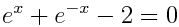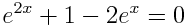First, multiply through by e^x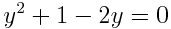Next, let y=e^x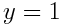Then just factor or use the quadratic formula.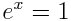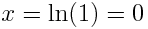Back substitute for y.

This technique is one which is often forgotten in favor of simply covering more new material, though it is extremely useful in solving a variety of difficult equations, in the same way that differentiating under the integral sign is ignored despite being useful for solving many otherwise near-impossible calculus problems. If you’re teaching algebra, try introducing it to your students by first asking them to solve an equation like the following: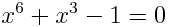To them, this equation will probably seem difficult, but substitution is certainly the most natural way to solve such a problem since it does not immediately lend itself to factoring.

One Response to Algebra Mini-Series #3: Using Substitution To Solve Equations

1. Pingback: Algebra Tricks – Solving Equations Using Substitution | Calcblog | Solve Math & Science Problems - Solveable.com